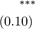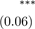# We Helped With This R Programming Assignment: Have A Similar One?

SOLVEDCategory Programming R | R Studio College Solved Statistic Homework Help

## Short Assignment Requirements

Please answer and write code for all the questions that attached in the file.

## Assignment Description

Exam12018

Mark F Owens

September 26, 2018

### Instructions

Please start with this exam template and create a new file in R Markdown called “exam1_your_name” to answer the following questions. Please change the name of the author as well. The questions in Part 1 do not require use of R, but you can type your answers into this R Markdown file if you would like. Part 2 requires you to use R. When you are finished, please knit the file and upload it to Canvas.

### Part 1 (40 Total Points)

1. Suppose you want to investigate the factors which predict a student’s grade on the econometrics exam.

(a)     Which factors that your instructor can observe do you think are important? (3 points)

(b)     Are there other important factors that your instructor cannot observe? (3 points)

2. Suppose you have daily sales data and weather reports from 2000-2018 for a restaurant. You want to determine if sales are related to the number of sunny days.

(a)     What kind of data do you have? (2 points)

(b)     Which is the dependent variable and which is the independent variable in this relationship? Briefly explain.(3 points)

3.     Specifically, what does it mean to have a coefficient with a p-value such that p<0.05? (3 points)

4.     Let colgpa denote the grade point average for a college student and let hsgpa denote the student’s gpa in high school. A simple model relating college gpa to high school gpa is

colgpa = β0 + β1hsgpa + µ,

in which µ represents the unobserved error.

(a)     What factors are contained in µ? (3 points)

(b)     Would you expect sign of βˆ1 to be positive, negative, or zero? Explain your answer. (3 points)

(c)     What characteristics would the hsgpa variable need to have in order for this simple regression model to uncover the “ceteris paribus” causal effect of hsgpa on colgpa? Explain. (3 points)

5. You are given the following table of output pertaining to voters in a sample of urban voting districts across the country. Voters is the number of voters who cast a ballot in 100s, and TV ads is the number of political advertisements purchased for TV in the market.Dependent variable:

 Voters TV Ads 1.25Constant                                                20.6(a)     Use the information in the table to write the regression equation. (3 points)

(b)     According to the results, how many voters are generated from each TV advertisement? (3 points)

(c)     How much of the variation in voters is explained by this model? Interpret this finding. (3 points)

(d)     Suppose one of the voting districts is Erie. There are 30 TV ads in Erie, and 6000 voters. Use the output in the table to calculate the predicted number of voters. (2 points)

(e)     What is the residual for Erie? (2 points)

(f)      Did Erie have more or fewer voters than would be expected for the number of TV Ads? (2 points)

(g)     What other factors would enter into the residual of this regression? (2 points)

Part 2: Data questions using R (60 Total Points)

Step 1: load the data (10 points)

### Step 2: Summary statistics (20 points)

Use R to calculate the following summary statistics.

(a)     What are the mean, median, minimum, and maximum score for exam1 for the entire sample? (1 point)

(b)     What is the standard deviation of exam1 for the entire sample? (1 point)

(c)     What are the mean, median, minimum, and maximum score for exam2 for the entire sample? (1 point) (d) What is the standard deviation of exam2 for the entire sample? (1 point)

(e)       What are the mean, median, minimum, and maximum score for finalexam for the entire sample? (1 point)

(f)        What is the standard deviation of finalexam for the entire sample? (1 point)

(g)       Create a histogram for exam1 for the entire sample. Label the figure. (2 points)

(h)       Create a kernel density plot for exam1 for the entire sample. Label the figure. (2 points)

(i)         Create a histogram for exam2 for the entire sample. Label the figure. (2 points)

(j)         Create a kernel density plot for exam2 for the entire sample. Label the figure. (2 points)

(k)       Create a histogram for finalexam for the entire sample. Label the figure. (2 points)

(l)         Create a kernel density plot for finalexam for the entire sample. Label the figure. (2 points)

(m)     Create a scatter plot with exam1 on the horizontal axis and finalexam on the vertical axis. Add a linear trend. Label the figure. (2 points)

### Step 3 (25 points)

Now use R to create new variables.

(a)     Create a new variable called overallgrade which takes the average of quiz, exam1, exam2, and finalexam and include it in your data frame.(3 points)

(b)     What are the mean, median, minimum, and maximum of overallgrade. (1 point)

(c)     What are the mean, median, minimum, and maximum of overallgrade for students who scored 80 or more on exam1? (3 points)

(d)     Create a new variable called woverallgrade which takes a weighted average of quiz, exam1, exam2, and finalexam and include it in your data frame. The weights are quiz 10%, exam1 25%, exam2 25%, and finalexam 40%. (3 points)

(e)     What are the mean, median, minimum, and maximum of woverallgrade. (1 point)

(f)      Perform a t-test of whether overallgrade is equal to woverallgrade. According to the t-test, are the two measures of overall grade different in a statistical sense? (3 points)

(g)     Perform a t-test of whether overallgrade is equal to exam1. According to the t-test, could we just use the score on exam 1 to assign an overall grade? (3 points)

(h)     Create a scatter plot with exam1 on the horizontal axis and overallgrade on the vertical axis. Add a linear trend. Label the figure. (3 points)

(i)       Create a new scatter plot with exam1 on the horizontal axis and overallgrade on the vertical axis, but do so only for students who scored 80 or higher on exam1 and exam2. Add a linear trend. Label the figure. (5 points)

### Part 4: Knit your file as .docx (5 points)

Knit your file as in docx format. (5 points)

Is it free to get my assignment evaluated?

Yes. No hidden fees. You pay for the solution only, and all the explanations about how to run it are included in the price. It takes up to 24 hours to get a quote from an expert. In some cases, we can help you faster if an expert is available, but you should always order in advance to avoid the risks. You can place a new order here.

How much does it cost?

The cost depends on many factors: how far away the deadline is, how hard/big the task is, if it is code only or a report, etc. We try to give rough estimates here, but it is just for orientation (in USD):

 Regular homework \$20 - \$150 Advanced homework \$100 - \$300 Group project or a report \$200 - \$500 Mid-term or final project \$200 - \$800 Live exam help \$100 - \$300 Full thesis \$1000 - \$3000

How do I pay?

Credit card or PayPal. You don't need to create/have a Payal account in order to pay by a credit card. Paypal offers you "buyer's protection" in case of any issues.

Why do I need to pay in advance?

We have no way to request money after we send you the solution. PayPal works as a middleman, which protects you in case of any disputes, so you should feel safe paying using PayPal.

Do you do essays?

No, unless it is a data analysis essay or report. This is because essays are very personal and it is easy to see when they are written by another person. This is not the case with math and programming.

Why there are no discounts?

It is because we don't want to lie - in such services no discount can be set in advance because we set the price knowing that there is a discount. For example, if we wanted to ask for \$100, we could tell that the price is \$200 and because you are special, we can do a 50% discount. It is the way all scam websites operate. We set honest prices instead, so there is no need for fake discounts.

Do you do live tutoring?

No, it is simply not how we operate. How often do you meet a great programmer who is also a great speaker? Rarely. It is why we encourage our experts to write down explanations instead of having a live call. It is often enough to get you started - analyzing and running the solutions is a big part of learning.

What happens if I am not satisfied with the solution?

Another expert will review the task, and if your claim is reasonable - we refund the payment and often block the freelancer from our platform. Because we are so harsh with our experts - the ones working with us are very trustworthy to deliver high-quality assignment solutions on time.

Customer Feedback

"Thanks for explanations after the assignment was already completed... Emily is such a nice tutor! "

Order #13073